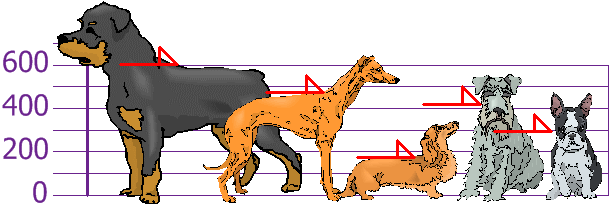# Mean Deviation

How far, on average, all values are from the middle.

## Calculating It

Find the mean of all values ... use it to work out distances ... then find the mean of those distances!

In three steps:

• 1. Find the mean of all values
• 2. Find the distance of each value from that mean (subtract the mean from each value, ignore minus signs)
• 3. Then find the mean of those distances

Like this:

### Example: the Mean Deviation of 3, 6, 6, 7, 8, 11, 15, 16

Step 1: Find the mean:

Mean =  3 + 6 + 6 + 7 + 8 + 11 + 15 + 168  =  728  = 9

Step 2: Find the distance of each value from that mean:

Value Distance from 9
3 6
6 3
6 3
7 2
8 1
11 2
15 6
16 7

Which looks like this:(No minus signs!)

Step 3. Find the mean of those distances:

Mean Deviation =  6 + 3 + 3 + 2 + 1 + 2 + 6 + 78  =  308  = 3.75

So, the mean = 9, and the mean deviation = 3.75

It tells us how far, on average, all values are from the middle.

In that example the values are, on average, 3.75 away from the middle.

For deviation just think distance

## Formula

The formula is:

Mean Deviation = Σ|x − μ|N

• Σ is Sigma, which means to sum up
• || (the vertical bars) mean Absolute Value, basically to ignore minus signs
• x is each value (such as 3 or 16)
• μ is the mean (in our example μ = 9)
• N is the number of values (in our example N = 8)

Let's look at those in more detail:

## Absolute Deviation

Each distance we calculate is called an Absolute Deviation, because it is the Absolute Value of the deviation (how far from the mean).To show "Absolute Value" we put "|" marks either side like this: |-3| = 3 For any value x: Absolute Deviation = |x - μ|

From our example, the value 16 has Absolute Deviation = |x - μ| = |16 - 9| = |7| = 7

And now let's add them all up ...

## Sigma

The symbol for "Sum Up" is Σ (called Sigma Notation), so we have:

Sum of Absolute Deviations = Σ|x - μ|

Divide by how many values N and we have:

Mean Deviation = Σ|x − μ| N

Let's do our example again, using the proper symbols:

### Example: the Mean Deviation of 3, 6, 6, 7, 8, 11, 15, 16

Step 1: Find the mean:

μ =  3 + 6 + 6 + 7 + 8 + 11 + 15 + 168  =  728  = 9

Step 2: Find the Absolute Deviations:

x |x - μ|
3 6
6 3
6 3
7 2
8 1
11 2
15 6
16 7
Σ|x - μ| = 30

Step 3. Find the Mean Deviation:

Mean Deviation =  Σ|x - μ|N  =  308  = 3.75

Note: the mean deviation is sometimes called the Mean Absolute Deviation (MAD) because it is the mean of the absolute deviations.

## What Does It "Mean" ?

Mean Deviation tells us how far, on average, all values are from the middle.

Here is an example (using the same data as on the Standard Deviation page):

### Example: You and your friends have just measured the heights of your dogs (in millimeters):The heights (at the shoulders) are: 600mm, 470mm, 170mm, 430mm and 300mm.

Step 1: Find the mean:

μ =  600 + 470 + 170 + 430 + 3005  =  19705  = 394

Step 2: Find the Absolute Deviations:

x |x - μ|
600 206
470 76
170 224
430 36
300 94
Σ|x - μ| = 636

Step 3. Find the Mean Deviation:

Mean Deviation =  Σ|x - μ|N  =  6365  = 127.2

So, on average, the dogs' heights are 127.2 mm from the mean.

(Compare that with the Standard Deviation of 147 mm)

## A Useful Check

The deviations on one side of the mean should equal the deviations on the other side.

From our first example:

### Example: 3, 6, 6, 7, 8, 11, 15, 16

The deviations are:6 + 3 + 3 + 2 + 1 = 2 + 6 + 7 15 = 15

Likewise:

### Example: Dogs

Deviations left of mean: 224 + 94 = 318

Deviations right of mean: 206 + 76 + 36 = 318

If they are not equal ... you may have made a msitake!